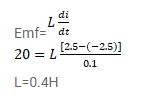# An average emf of 20V is induced in an inductor

Question:

An average emf of $20 \mathrm{~V}$ is induced in an inductor when the current in it is changed from $2.5 \mathrm{~A}$ in one direction to the same value in the opposite direction in $0.1 \mathrm{~s}$. Find the self-inductance of the inductor.

Solution: